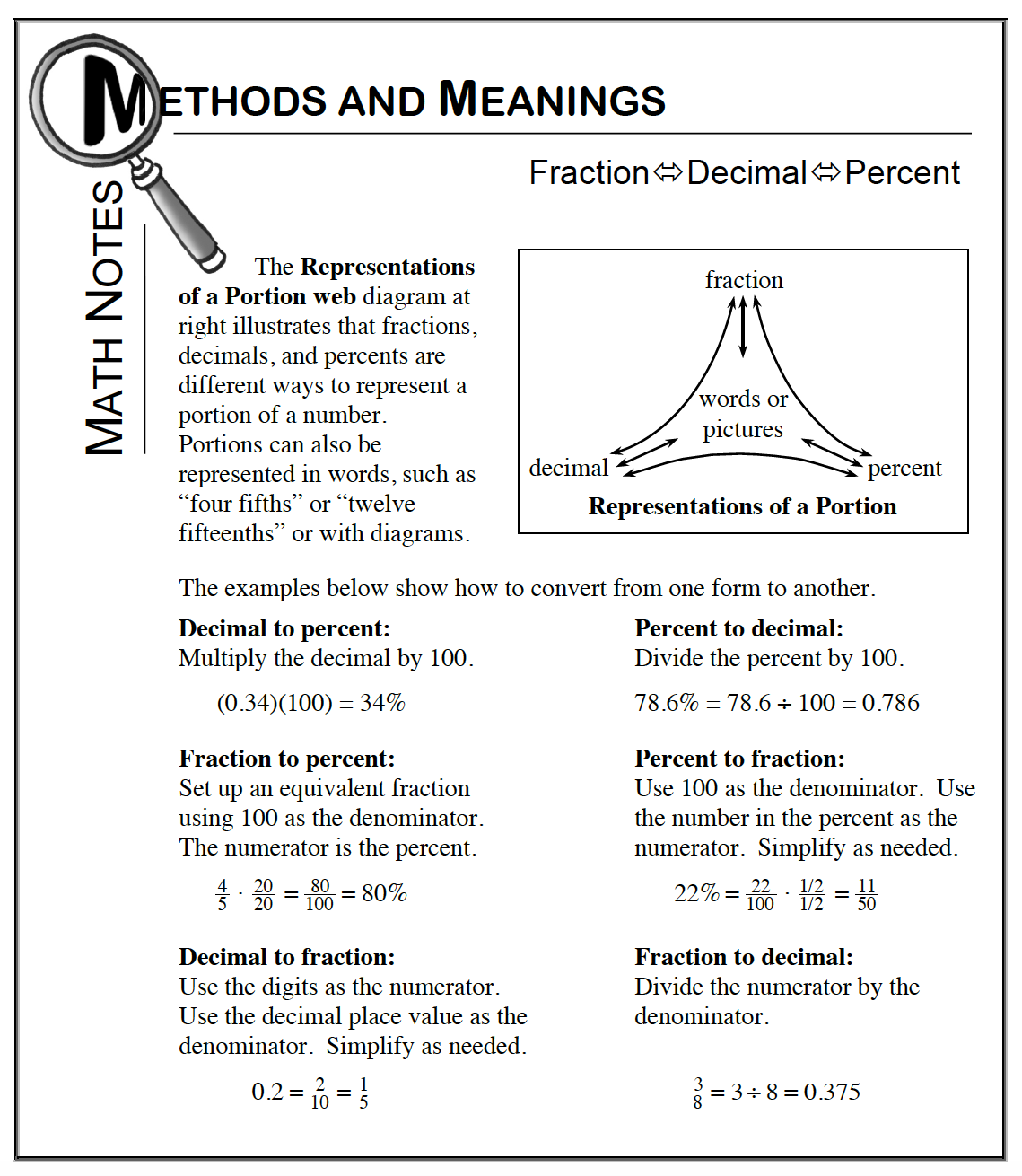Home > CC1 > Chapter 7 > Lesson 7.1.2 > Problem7-21

7-21.

Complete the Representations of a Portions web for each of the following portions. Show your work so that a team member could understand your process. Homework Help ✎

1. $\frac { 3 } { 5 }$

• Refer to the Math Notes box from Lesson 3.1.5 at the bottom of this page.

1. $0.7$

• Refer to part (a).

• $\frac{7}{10}\ \text{and}\ 70\%$

1. $16 \%$

• Refer to part (a).

$0.16\ \text{and}\ \frac{4}{25}$

1. $2.45$

• Refer to part (a).

$245\%\ \text{and}\ 2\frac{9}{20}$